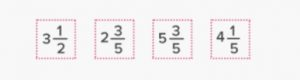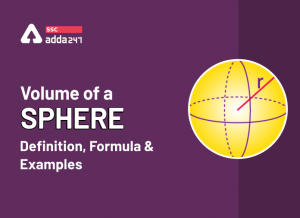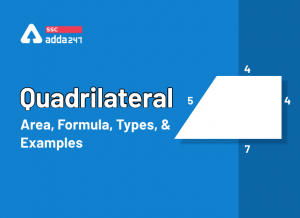Latest SSC jobs   »   Fractions   »   Fractions

# What is Fraction? – Definition, Examples, Addition, Calculator, Multiplication

Fractions: A word fraction originated from the Latin word “fraction” which means “to break” as fraction means to break or divide a fixed part from the whole. A fraction is defined as a part of a whole thing i.e. fraction is not a whole number while it’s a part of a whole number that acquires a fixed part of it. A fraction is used to represent a part of the whole thing. It is expressed in form of numerator and denominator.

## Fractions Definition

Simply a fraction is represented in x/y form where x is a numerator which is shown at the top and y is a denominator shown below. It means x is a whole thing and y is some fixed part of x. In our daily life, we deal with fractions many times like we take 1/2 tumbler of water, 1/4 mug of syrup, 1/2 part of chappati, etc.

The daily life examples of fractions are listed below:

• If a cake is divided into four equal parts by cutting then every four parts are equal to a whole cake.
• If we divide an apple into three equal parts then every part will be equal to 1/3 of the apple.

## Fraction Types

A fraction is a ratio or part of a whole quantity. It is represented in the form of numerator and denominator. Based on the numerator and denominator  fractions are of the following types:

• Proper Fractions
• Improper Fractions
• Mixed Fractions
• Like Fractions
• Unlike Fractions
• Equivalent Fractions
• Unit Fractions

All these types of fractions are discussed in detail with examples below. So refer to the below content to know about all such types of fractions in detail.

Proper Fractions

A fraction having a numerator less than the denominator is known as a proper fraction. It is identified by simply comparing the values of the numerator and denominator. For example 3/7, 4/5, 2/5, 8/9, 1/4, 2/7, and so on where the numerator is less than the denominator.

Improper Fractions

A fraction whose numerator is greater than or equal to the denominator is known as an improper fraction. It is just the opposite of proper fractions and can be identified by observing the values of the numerator and denominator. For example 3/2, 7/4, 8/5, 4/3, 9/2, 6/5, 7/6, and so on.

Mixed Fractions

A mixed fraction is a combination of a whole number and a fraction. It is an improper fraction. It turned on combining a whole number and a proper fraction. It can be simplified on performing mathematical operations. For exampleThe mixed fractions are shown above.

Like Fractions

A fraction whose denominator is the same is called a like fraction. The denominator of like fractions remains the same always. For example, 2/5, 3/5, 4/5, 6/5, 7/5, 8/5, 19/5, 23/5, and so on whose denominator is the same i.e. 5.

The simplification of like can be done easily using basic mathematical operations.

Unlike Fractions

A fraction whose numerator is the same is called, unlike fractions. The denominator can be different but the numerator remains the same value. For example 1/2, 3/2, 5/2, 7/2, 9/2, 11/2, and 13/2, are unlike fractions.

## Equivalent Fractions

The fractions that represent the same values after simplification are known as equivalent fractions. The fractions are equal to each other and termed equivalent fractions. For example, 2/4 and 8/16, 3/9, and 18/36 are equivalent fractions.

Unit Fractions

The fractions have a numerator 1 and the denominator has a positive integer called unit fractions. For example, 1/2, 1/3, 1/4, 1/5, and 1/6 are unit fractions.

Equivalent Fractions

Equivalent fractions are the fractions whose generators and denominators both are different but they give the same value on simplification. Equivalent fractions can be defined as when two or more fractions give the same value on simplification are equivalent fractions to each other. An equivalent fraction is a group of fractions that represents the same value on simplification.

Fraction equivalent gives the same value on amplification. Fraction equivalent is a property of fraction that represents the same value after solving. Fraction equivalent gives always the same value on applying mathematical operations.

For example, 1/4 and 25/100, 3/4 and 12/16, 9/27, and 8/24 are equivalent fractions to each other.

## Decimal to Fraction

Decimals can be converted to fractions easily using simple mathematical steps. Decimal to fraction conversion removes a decimal point and gives a value in a fraction form. Decimal to fraction can be converted using the steps listed below.

• First write the given decimal in the fraction form (x/y), where the denominator should be 1.
• Then multiply the numerator and denominator by multiples of 10, counting the decimal position, such that the decimal in the numerator becomes a whole number. (If there are two numbers after the decimal point, then multiply by 100/100, and 1000.1000 if three numbers after the decimal)
• Further, simplify the obtained fraction if required

For example, Convert 0.25 to fraction form

Given fraction, 0.25

Where decimal is placed after two numbers then we will multiply by 100/100 in both numerator and denominator to get the required fraction.

0.25/100×100 = 1/4

So 1/4 is a required fraction obtained from 0.25

Fractions addition is a method to add two or more fractions to get a combined fraction. Fraction addition can be done by performing simple mathematical operations as an addition in algebraic expressions. Fraction addition represents a combined resultant fraction on addition.

The addition of fractions can be done simply as we add numbers but in fraction addition, we need to check the values of the denominator. The addition of fractions can be simply done if has the same denominator but has a different denominator the addition requires some different steps to add the fractions. The solved examples of fraction addition are mentioned here for the ease of students.

Fraction Addition with the Same Denominator

If the denominators of two or more fractions are the same, then we can directly add the numerators, keeping the denominator common.

Follow the below-mentioned steps to add the fractions with the same denominators:

• Add the numerators together, keeping the denominator common
• Get the simplified fraction

Example: Add the fractions: 5/6 and 7/6.

Solution: Since the denominators are the same, therefore we can add the numerators directly keeping the denominator common

(5/6) + (7/6) = (5 + 7)/6 = 12/6

On simplifying fraction

12/6 = 2

Hence, the required sum of fractions ⅚ and 7/6 is 2.

When the denominators of the given fractions are different then we can not add numerators directly by keeping the denominator common. On adding the fractions with different denominators we need to follow the LCM method as listed below.

• Firstly, Check the denominators of the given fractions.
• Make the denominators of the fractions the same, by taking the LCM’s other denominators and then rationalizing them
• Add the numerators of the fractions simply keeping the denominator common
• Simplify the obtained fraction to get the final sum

Example: Add the fractions 3/15 + 5/2

Solution: Both the given fractions 3/15 and 5/2 have different denominators.

We can write 3/15 = 1/5, in a simplified fraction.

Now, 1/5 and 5/2 are two fractions.

LCM of 2 and 5 = 10

Multiply 5/2 by 1/5, then

The required fraction is 27/10

## Fraction Calculator

A fractions calculator is a method to reduce a complex or big fraction into a simplified fraction form. The fraction calculator gives a required small fraction value. The students can also use a fraction calculator tool to solve a fraction. Calculator fraction gives a small value in simplified form by performing mathematical operations.

## Fraction Multiplication

Fractions multiplication is a product of two fractions to get a single resultant fraction. Fraction multiplication is initiated by multiplying the numerators followed by denominators. The multiplication of fractions can be done by multiplying simply numerators and denominators. The multiplication of fractions can be performed by following the steps listed below.

• First, multiply the numerators.
• Then multiply the denominators.
• Reduce the resultant fraction to its lowest terms.

Example: Multiply the fractions 1/5 and 3/4

Solution: 1/5 and 3/4

Multiply the numerators then denominators we get

= 3/20

So the resultant multiplication of 1/5 and 3/4 is 3/20.

## Fraction: FAQs

Que.1 What is a fraction?

Ans – A fraction is a part of the whole quantity represented in the form x/y. For example, 2/5 is a fraction.

Que.2 What is a proper fraction?

Ans- A fraction having a numerator less than the denominator is called a proper fraction. For example 1/4.

Que.3 What are equivalent fractions?

Ans – When two fractions give the same value on simplification then both are equivalent fractions. For example 3/9 and 4/12.

Que.4 Multiply the fractions 2/3 and 4/9

Solution: 2/3 and 4/9

On multiplying numerators and denominators we get 8/27

So the multiplication of 2/3 and 4/9 gives a fraction of 8/27.

#### Congratulations!General Awareness & Science Capsule PDF•Comprehension Passage in English, Readin...
•What is Frequency Polygon? Statistics, G...
•Volume of a Sphere
•Quadrilateral Area, Formula, Types, Prop...